Prateek Joshi — Published On August 4, 2020 and Last Modified On August 4th, 2020

## Overview

• Introduction to Natural Language Generation (NLG) and related things-
• Data Preparation
• Training Neural Language Models
• Build a Natural Language Generation System using PyTorch

## Introduction

In the last few years, Natural language processing (NLP) has seen quite a significant growth thanks to advancements in deep learning algorithms and the availability of sufficient computational power. However, feed-forward neural networks are not considered optimal for modeling a language or text. This is because the feed-forward network does not take into consideration the word order in the text.Hence, to capture the sequential information present in the text, recurrent neural networks are used in NLP. In this article, we will see how we can use a recurrent neural network (LSTM), using PyTorch for Natural Language Generation.

If you need a quick refresher on PyTorch then you can go through the article below:

And if you are new to NLP and wish to learn it from scratch, then check out our course:

1. A Brief Overview of Natural Language Generation (NLG)
2. Text Generation using Neural Language Modeling
3. Understanding the Functioning of Neural Language Models
– Data Preparation
– Model Training
– Text Generation
4. Natural Language Generation using PyTorch

## A Brief Overview of Natural Language Generation

Natural Language Generation (NLG) is a subfield of Natural Language Processing (NLP) that is concerned with the automatic generation of human-readable text by a computer. NLG is used across a wide range of NLP tasks such as Machine Translation, Speech-to-text, chatbots, text auto-correct, or text auto-completion.

We can model NLG with the help of Language Modeling. Let me explain the concept of language models – A language model learns to predict the probability of a sequence of words. For example, consider the sentences below: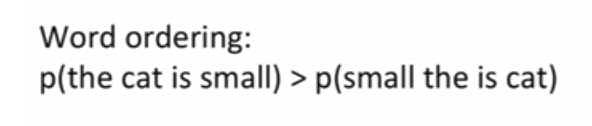We can see that the first sentence, “the cat is small”, is more probable than the second sentence, “small the is cat”, because we know that the sequence of the words in the second sentence is not correct. This is the fundamental concept behind language modeling. A language model should be able to distinguish between a more probable and a less probable sequence of words (or tokens).

### Types of Language Models

The following are the two types of Language Models:

1. Statistical Language Models: These models use traditional statistical techniques like N-grams, Hidden Markov Models (HMM), and certain linguistic rules to learn the probability distribution of words.
2. Neural Language Models: These models have surpassed the statistical language models in their effectiveness. They use different kinds of Neural Networks to model language.

In this article, we will focus on RNN/LSTM based neural language models. If you want a quick refresher on RNN or LSTM then please check out these articles:

## Text Generation using Neural Language Modeling

### Text Generation using Statistical Language Models

First of all let’s see how we can generate text with the help of a statistical model, like an N-Gram model. To understand how an N-Gram language model works then do check out the first half of the below article:

Suppose we have to generate the next word for the below sentence: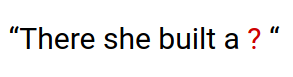Let’s say that our N-Gram model considers a context of 3 previous only to predict the next word. So, the model will try to maximize the probability P(w | “she built a”), where ‘w’ represents each and every word in the text dataset. Whichever word that maximizes that probability will be generated as the next word for the sentence “There she built a…”.

However, there are certain drawbacks of using such statistical models that use the immediate previous words as context to predict the next word. Let me give you some extra context.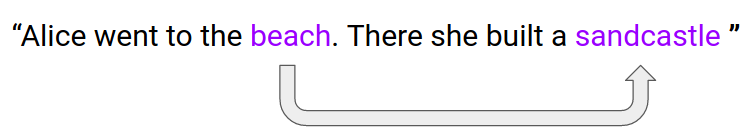Now we have some more information about what’s going on. The term “sandcastle” is very likely as the next word because it has a strong dependency on the term “beach” because people build sandcastles on beaches mostly right. So, the point is that “sandcastle” does not depend on the immediate context (“she built a”) as much as it depends on “beach”.

### Text Generation using Neural Language Models

To capture such unbounded dependencies among the tokens of a sequence we can use an RNN/LSTM based language model. The following is a minimalistic representation of the language model that we will use for NLG: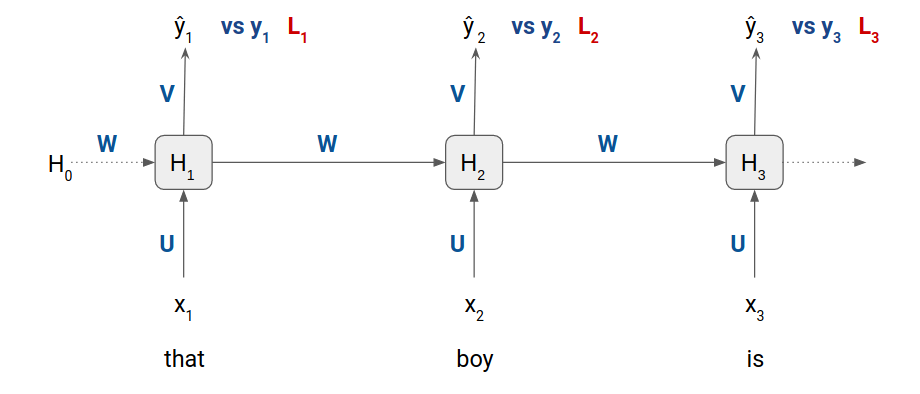• x1, x2, and x3 are the inputs word embeddings at timestep 1, timestep 2, and timestep 3 respectively
• ŷ1, ŷ2, and ŷ3 are the probability distribution of all the distinct tokens in the training dataset
• y1, y2, and y3 are the ground truth values
• U, V, and W are the weight matrices
• and H0, H1, H2, and H3 are the hidden states

We will cover the working of this neural language model in the next section.

## Understanding the Functioning of Neural Language Models

We will try to understand the functioning of a neural language model in three phases:

• Data Preparation
• Model Training
• Text Generation

### 1. Data Preparation

Let’s assume that we will use the sentences below as our training data.

[ ‘alright that is perfect’,
‘that sounds great’,
‘what is the price difference’]

The first sentence has 4 tokens, the second has 3 and the third has 5 tokens. So, all these sentences have varying lengths in terms of tokens. An LSTM model accepts sequences of the same length only as inputs. Therefore, we have to make the sequences in the training data have the same length.

There are multiple techniques to make sequences of equal length.

One technique is padding. We can pad the sequences with padding tokens wherever required. However, if we use this technique then we will have to deal with the padding tokens during loss calculation and text generation.

So, we will use another technique that involves splitting a sequence into multiple sequences of equal length without using any padding token. This technique also increases the size of the training data. Let me apply it to our training data.

Let’s say we want our sequences to have exactly three tokens. Then the first sequence will be split into the following sequences:

[ ‘alright that is’,
‘that is perfect’ ]

The second sequence is of length three only so it will not be split. However, the third sequence of the training data has five tokens and it will be broken down into multiple sequences of tokens:

[ ‘what is the’,
‘is the price’,
‘the price difference’ ]

Now the new dataset will look something like this:

[ ‘alright that is’,
‘that is perfect’,
‘that sounds great’,
‘what is the’,
‘is the price’,
‘the price difference’ ]

### 2. Model Training

Since we want to solve the next word generation problem, the target should be the next word to the input word. For example, consider the first text sequence “alright that is”.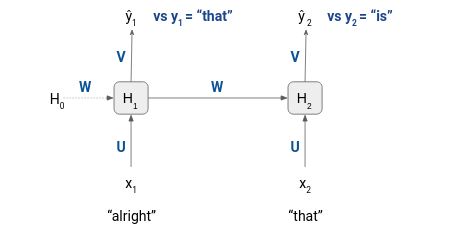As you can see, with respect to the first sequence of our training data, the inputs to the model are “alright” and that”, and the corresponding target tokens are “that” and “is”. Hence, before starting the training process, we will have to split all the sequences in the dataset to inputs and targets as shown below: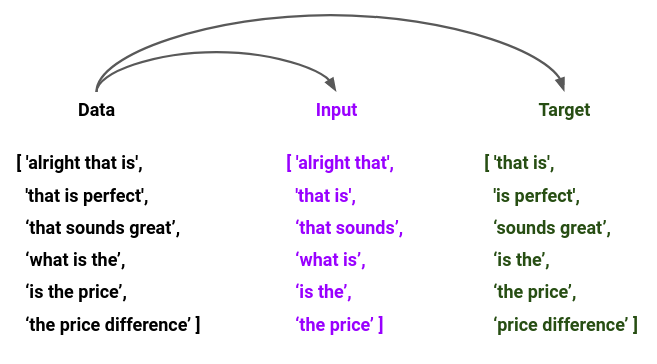So, these pairs of sequences under Input and Target are the training examples that will be passed to the model, and the loss for a training example will be the mean of losses at each timestep.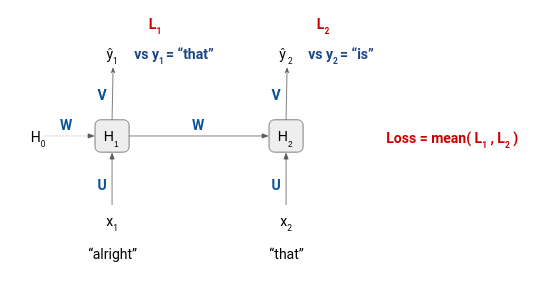Let’s see how this model can then be used for text generation.

### 3. Text Generation

Once our language model is trained, we can then use it for NLG. The idea is to pass a text string as input along with a number of tokens you the model to generate after the input text string. For example, if the user passes “what is” as the input text and specifies that the model should generate 2 tokens, then the model might generate “what is going on” or “what is your name” or any other sequence.

Let me show you how it happens with the help of some illustrations:

input text = “what is”
n = 2

Step 1 – The first token (“what”) of the input text is passed to the trained LSTM model. It generates an output ŷ1 which we will ignore because we already know the second token (“is”).  The model also generates the hidden state H1 that will be passed to the next timestep.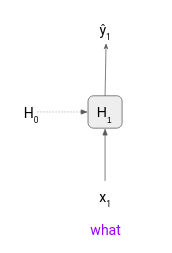Step 2 – Then the second token (“is”) is passed to the model at timestep 2 along with H1. The output at this timestep is a probability distribution in which the token “going” has the maximum value. So, we will consider it as the first generated or predicted token by our model. Now we have one more token left to generate.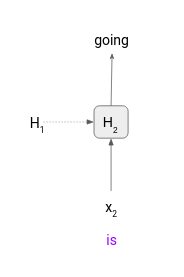Step 3 – In order to generate the next token we need to pass an input token to the model at timestep 3. However, we have run out of the input tokens, “is” was the last token that generated “going”. So, what do we pass next as input? In such a case we will pass the previously generated token as the input token.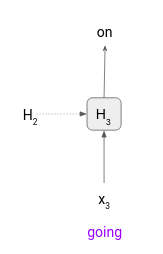The final output of the model would be “what is going on”. That is the text generation strategy that we will use to perform NLG. Next, we will train our own language model on a dataset of movie plot summaries.

## Natural Language Generation using PyTorch

Now that we know how a neural language model functions and what kind of data preprocessing it requires, let’s train an LSTM language model to perform Natural Language Generation using PyTorch. I have implemented the entire code on Google Colab, so I suggest you should use it too.

Let’s quickly import the necessary libraries.

We will work with a sample of the CMU Movie Summary Corpus. You can download the pickle file of the sample data from this link.

You can use the code below to print five summaries, sampled randomly.

```# sample random summaries
random.sample(movie_plots, 5)```

### 2. Data Preparation

First of all, we will clean our text a bit. We will keep only the alphabets and the apostrophe punctuation mark and remove the rest of the other elements from the text.

```# clean text
movie_plots = [re.sub("[^a-z' ]", "", i) for i in movie_plots]```

It is not mandatory to perform this step. It is just that I want my model to focus only on the alphabet and not worry about punctuation marks or numbers or other symbols.

Next, we will define a function to prepare fixed-length sequences from our dataset. I have specified the length of the sequence as five. It is a hyperparameter, you can change it if you want.

So, we will pass the movie plot summaries to this function and it will return a list of fixed-length sequences for each input.

Output: 152644

Once we have the same length sequences ready, we can split them further into input and target sequences.

Now we have to convert these sequences (x and y) into integer sequences, but before that, we will have to map each distinct word in the dataset to an integer value. So, we will create a token to integer dictionary and an integer to the token dictionary as well.

Output: (14271, ‘the’)

```# set vocabulary size
vocab_size = len(int2token)
vocab_size```

Output: 16592

The size of the vocabulary is 16,592, i.e., there are over 16,000 distinct tokens in our dataset.

Once we have the token to integer mapping in place then we can convert the text sequences to integer sequences.

### 3. Model Building

We will pass batches of the input and target sequences to the model as it is better to train batch-wise rather than passing the entire data to the model at once. The following function will create batches from the input data.

Now we will define the architecture of our language model.

The input sequences will first pass through an embedding layer, then through an LSTM layer. The LSTM layer will give a set of outputs equal to the sequence length, and each of these outputs will be passed to a linear (dense) layer on which softmax will be applied.

Output:

WordLSTM(
(emb_layer): Embedding(16592, 200)
(lstm): LSTM(200, 256, num_layers=4, batch_first=True, dropout=0.3)
(dropout): Dropout(p=0.3, inplace=False)
(fc): Linear(in_features=256, out_features=16592, bias=True)
)

Let’s now define a function that will be used to train the model.

```# train the model
train(net, batch_size = 32, epochs=20, print_every=256)```

I have specified the batch size of 32 and will train the model for 20 epochs. The training might take a while.

### 4. Text Generation

Once the model is trained, we can use it for text generation. Please note that this model can generate one word at a time along with a hidden state. So, to generate the next word we will have to use this generated word and the hidden state.

The function sample( ) takes in an input text string (“prime”) from the user and a number (“size”) that specifies the number of tokens to generate. sample( ) uses the predict( ) function to predict the next word given an input word and a hidden state. Given below are a few text sequences generated by the model.

`sample(net, 15)`

#### Output:

‘it is now responsible by the temple where they have the new gospels him and is held’

`sample(net, 15, prime = "one of the")`

#### Output:

‘one of the team are waiting by his rejection and throws him into his rejection of the sannokai’

`sample(net, 15, prime = "as soon as")`

#### Output:

‘as soon as he is sent not to be the normal warrior caused on his mouth he has’

`sample(net, 15, prime = "they")`

#### Output:

‘they find that their way into the ship in his way to be thrown in the’

## End Notes

Natural Language Generation is a rapidly maturing field and increasingly active field of research. The methods used for NLG have also come a long way from N-Gram models to RNN/LSTM models and now transformer-based models are the new state-of-the-art models in this field.

To summarize, in this tutorial, we covered a lot of things related to NLG such as dataset preparation, how a neural language model is trained, and finally Natural Language Generation process in PyTorch. I suggest you try to build a language model on a bigger dataset and see what kind of text it generates.

In case you are looking for a roadmap to becoming an expert in NLP read the following article-

Please feel free to comment below if you have any queries.###### Prateek Joshi

Data Scientist at Analytics Vidhya with multidisciplinary academic background. Experienced in machine learning, NLP, graphs & networks. Passionate about learning and applying data science to solve real world problems.